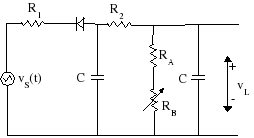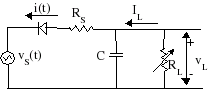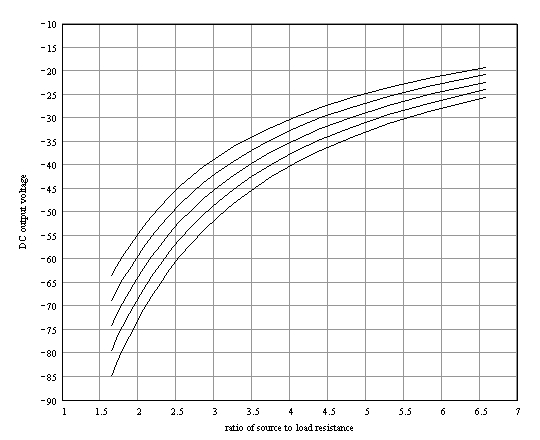The figure below shows the Marshall JMP50 Model 1987 grid bias supply circuit. The bias voltage VL is applied to the EL34 pentode control grids, which under zero-signal conditions draw no current and represent an open circuit.The JMP50 power transformer has a high-voltage output described as 350-0-350, indicating that it has a center tap (normally grounded) and two, 350-volt RMS outputs of opposite phase. (An RMS output of 350 volts corresponds to a sinewave with an amplitude of 495 volts.) The high voltage is rectified and filtered to provide a DC high-voltage supply to the tube plates and the pentode screens. One of these phases also powers the EL34 grid bias circuit. The Model 1987 circuit values are

R1 = 220k
R2 = 15k
RA = 47k
RB = 25k
C = 8uF

The diode rectifies the AC input voltage and is oriented to pass only negative voltages. So the diode is a short circuit if vS(t) < VL and is an open circuit otherwise.

Silicon diodes are not perfect. When conducting there is a small voltage drop across the diode that increases with increasing current and when not conducting there is a small leakage current. For a typical glass-passivated 1N4007, for example, the forward voltage drop is 0.6 volts when conducting a current of 10 milliamps, increasing to 0.9 volts at a current of 1 amp. For comparison, if VL = 0 the maximum current possible across the JMP50 diode would be only 495/215k = 2.3mA. As the capacitors in the circuit charge, the maximum current will be even less. The leakage current of a 1N4007 is 5 microamps at room temperature, increasing to only 50 microamps at a temperature of 125 degrees Celsius. For the voltages and currents in the Marshall Model 1987 power supply all of these values are insignificant, so the diode can be considered perfect for the purposes of determining the JMP50 grid bias voltage adjustment range.

## Simplifying the Schematic

The JMP50 filter consists of two capacitors connected by R2 and is quite effective in eliminating AC ripple. This can be seen if we consider the time constant formed by just the load and the capacitor across it. The worst case would be for the variable resistor to be set to zero, giving a time constant of

RAC = (47k)(8uF) = 0.38 seconds

When vS(t) < VL the diode conducts and recharges the capacitors. When the diode cuts off the load continues to draw current, which is supplied by the upstream capacitor via R2 and the capacitor across the load. Now consider a worse situation: we remove the upstream capacitor from the JMP50 circuit. This gives the capacitor across the load no chance to recharge itself during the time period when the diode is in cutoff. The voltage across the load would then decay according to

VL(t) = V0exp(-t / 0.38)

where V0 is the voltage across the load at time t = 0 when the diode goes into cutoff. If the AC supply is at 50Hz (European power) we would expect the diode to start conducting again within 1/50 = 0.02 seconds. During this time period the voltage across the load would decay to

VL(0.02) = (0.95)V0

So the bias voltage would retain 95 percent of its initial value. With the additional upstream capacitor and with the variable resistor set to somewhere near midrange (reducing the current load), the voltage decay is much less. So there is very little ripple in the DC bias supply output and for computing the JMP50 bias voltage we can consider VL to be a constant. This greatly simplifies the mathematics involved. We can therefore use the following simplified schematic and still get accurate results.Here RS = R1 + R2 = 235k represents the total source resistance. (We omit the much smaller resistance in the transformer, which is about 75 ohms across each phase for a typical 350-0-350 secondary.) For the Marshall JMP50 Model 1987 the load resistance is in the range 47k < RL < 72k depending on the variable resistor setting. To emphasize that the output voltage is a constant, even when the diode is not conducting, we place an infinitely large capacitor C across the load. Notice that because of the orientation of the diode that the grid bias voltage VL is negative.

## Computing the Grid Bias Adjustment Range

The following graph shows the grid bias voltage versus the ratio RS / RL for the simplified grid bias supply schematic. The curves are for five different transformers: 300-0-300 (upper curve), 325-0-325, 350-0-350, 375-0-375, and 400-0-400 (lower curve).The ratio of source to load resistance for the Marshall JMP50 Model 1987 varies from 5 (variable resistor set to minimum) to 3.3 (resistor set to maximum). The middle curve, corresponding to Marshall's 350-0-350 power transformer, shows that the grid bias voltage can be adjusted between -28 and -42 volts. The ultimate adjustment range will vary depending on the error tolerance of the components and variations in AC line voltage.

## References

1Richard Kuehnel, Circuit Analysis of a Legendary Tube Amplifier: The Fender Bassman 5F6-A, 2nd Ed., (Seattle: Pentode Press, 2005).

2Michael Doyle, The History of Marshall, (Milwaukee: Hal Leonard Corp., 1993).